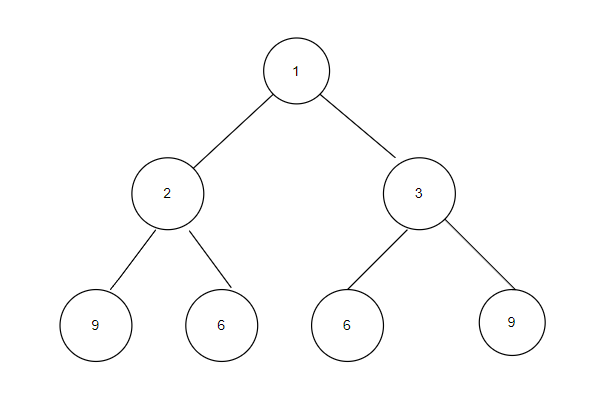# Palindromic Levels Of a Binary Tree

## Introduction

Palindrome and Level order traversal of a binary tree are two questions that are asked frequently in coding interviews, But what happens when you combine these two concepts? We get something that we call Palindromic Levels Of a Binary Tree in which we have to print only those levels which are palindromic.

## Understanding the Problem

We have been given a binary tree, and we have to print its level order traversal, But we only have to print those levels which are palindrome. This thing will become more clear by the following example.Now, if we print the level order traversal of the tree, we will get

``````1
2 3
9 6 6 9``````

According to the question, we only have to print those levels which are palindromic. Thus our output will be

``````1
9 6 6 9``````

## Intuition

The intuition is very straightforward, We’ll do a regular level order traversal using a queue, and after we have computed a particular level, we will check if it’s a palindrome or not. If it’s a palindrome, we will print it; otherwise, we will skip it.

Now let’s look at the code for the above algorithm.

### Code

``````#include <iostream>
#include <queue>

using namespace std;

// Binary Tree Node Class.
template <typename T>
class BinaryTreeNode
{
public:
T val;
BinaryTreeNode<T> *left;
BinaryTreeNode<T> *right;

BinaryTreeNode(T val)
{
this->val = val;
left = NULL;
right = NULL;
}
};

// Function to check palindrome.
bool isPalindrome(vector<int> v1)
{
for (int i = 0, j = v1.size() - 1; i < j; i++, j--)
{
if (v1[i] != v1[j])
return false;
}
return true;
}

void levelOrderPalindrome(BinaryTreeNode<int> *root)
{
// Queue for level order traversal.
queue<BinaryTreeNode<int> *> q1;
// Base Case.
if (root == NULL)
return;
q1.push(root);

while (q1.size() != 0)
{
// Vector to store level order traversal of each level.
vector<int> v1;
int s = q1.size();
while (s > 0)
{
BinaryTreeNode<int> *top = q1.front();
v1.push_back(top->val);

// Pushing its left and right node.
if (top->left != NULL)
q1.push(top->left);
if (top->right != NULL)
q1.push(top->right);
s--;
q1.pop();
}

// If the level is palindromic, then we will print it.
if (isPalindrome(v1))
{
for (int i = 0; i < v1.size(); i++)
cout << v1[i] << " ";
cout << endl;
}
}
}

BinaryTreeNode<int> *takeInput()
{
int rootData;
cin >> rootData;

if (rootData == -1)
{
return NULL;
}

BinaryTreeNode<int> *root = new BinaryTreeNode<int>(rootData);
queue<BinaryTreeNode<int> *> q;
q.push(root);

while (!q.empty())
{
BinaryTreeNode<int> *currentNode = q.front();
q.pop();
int leftChild, rightChild;

cin >> leftChild;
if (leftChild != -1)
{
BinaryTreeNode<int> *leftNode = new BinaryTreeNode<int>(leftChild);
currentNode->left = leftNode;
q.push(leftNode);
}

cin >> rightChild;
if (rightChild != -1)
{
BinaryTreeNode<int> *rightNode = new BinaryTreeNode<int>(rightChild);
currentNode->right = rightNode;
q.push(rightNode);
}
}
return root;
}

int main()
{
// Taking input.
BinaryTreeNode<int> *root = takeInput();

// Calling 'levelOrderPalindrome()' function.
levelOrderPalindrome(root);
}``````

### Input

``1 2 3 9 6 6 9 -1 -1 -1 -1 -1 -1 -1 -1``

Here we are taking input level order wise and -1 here represents a NULL node.The input tree would look something like this.

### Output

``````1
9 6 6 9``````

### Time Complexity

O(N), where ‘N’ is the number of nodes in the tree.

The level order traversal would cost O(N) time as each node is pushed exactly once in the queue.

Let us analyze the time complexity in terms of palindrome check. As the worst case will occur when the tree is a complete binary tree. Now for palindrome check, for the first level, it will take 2 ^ 0 time, for the second level it will take 2 ^ 1 time, for the third level it will take 2 ^ 2 time and so on. Thus the overall complexity would be the summation of 2 ^ 0 + 2 ^ 1 + 2 ^ 2 ……….2 ^ (log (N - 1)).

This is a GP with the first term, a = 1, and common ratio, r = 2.

Let the number of terms in this GP be k.

Then last term, 2 ^ (log(N - 1)) = a * r ^ (k - 1)

2 ^ (log(N - 1)) = 1 * 2 ^ (k - 1)

2 ^ (log(N - 1)) = 2 ^ (k - 1)

log(N - 1) = (k - 1)

k = log(N - 1) + 1

Hence, the sum of series = a * (r ^ k - 1) / (r - 1) = 1 * (2 ^ k - 1) / (2 - 1) = 2 ^ k - 1 = 2 ^ (log(N - 1) - 1 = log(N - 1) - 1

Overall time complexity = O(log(N) + O(N)) = O(N)

### Space Complexity

O(N), where 'N' is the number of nodes in the tree.

Since we are using a queue for level order traversal, it will take extra O(N) space.

## Key Takeaways

Now you have understood how to find the palindromic levels of a binary tree,  which refreshed your concepts about palindrome and level order traversal of Binary Tree. There are many variations to these two concepts, but you don’t have to worry about any of it when your ninja friend is here, so head over to our practice platform CodeStudio to practice top problems and many more. Till then, Happy Coding!